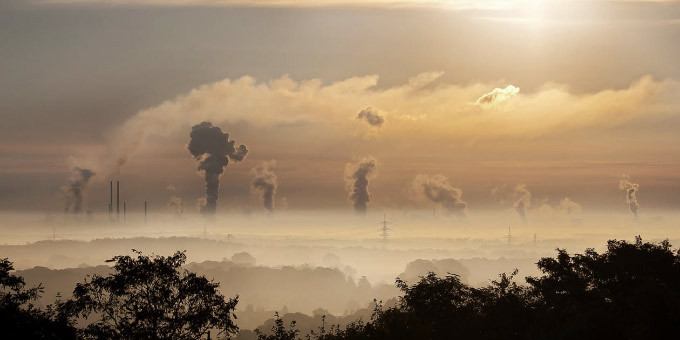# How much carbon is in the atmosphere?

Let’s start from the mass of the Earth atmosphere, which is 5.137 × 10^15 tonnes
(5.137 × 10^18 kg).

Mol is is defined as the amount of a chemical substance that contains as many representative particles (atoms, molecules, ions, electrons, or photons) as there are atoms in 12 grams of carbon-12; therefore, one mole has 12.011 g C, and 1 gram of carbon has 0.083257 mole.

We can calculate that 1 mole of CO2 has a weight of 44.0087 grams
(12.0107 (C) + 15.999 (O) + 15.999 (O)).If we take the highest measured concentration of CO2 as 410.28 ppmv, the percentage content would be equal to 0.041028%.

Now, we can calculate the following: 0.041028% (CO2 atmosphere volume) * 28.971 g/mole (average molar mass of dry air) / 44.0087 g (CO2). This will give us 0.0623240117 m% CO2.

Furthermore, multiplying Earth’s atmosphere 5.137 × 10^15 tonnes * 0.0623240117 m% CO2, we will see that the total weight of CO2 in the atmosphere is ~3,208 Gt CO2.

As the carbon content in carbon dioxide is 27.3%, that also means that each 1 ppmv (part per million of volume) of the atmosphere weighs approximately 2.1357 Gt of Carbon.

As I said, current CO2 levels have reached the dangerously high 410.28 ppmv, and it is said that if we reach 450ppmv there is a 50% chance that we will reach 2C, which many call the point of no return.

Let’s now consider how much CO2 or Carbon we have added since the Industrial Revolution and how much carbon we need to sequester, in order to reach what is considered the stable level of 350ppmv.

We will take the year 1800 as the comparison point for pre-industrial CO2 concentration in the atmosphere. At that time, the CO2 level was around 280 ppmv, or, in other words, since pre-industrial times, human influence has added 130.28, which equates to 278.24 Giga tonnes of Carbon ((410.28-280) * 2.1357 GtC per ppmv ).

In order to go back to the safe level of 350 ppmv, we would need to sequester 128.74 GtC
((410.28-350)*2.1357).

But, how much is that?

Saying 128.74 Giga-tonnes of carbon sounds quite abstract and does not mean much.

If we understand that there are 7.5 billion people on this planet, and we task each person to take care of his or her own portion, each person would need to sequester around 17,165 kg of Carbon.

If we would relate that amount to standard cement bags, each of us would need to take care of 687 (25 kg) bags. Amount enough to fill a 20-ft container truck!
Therefore, we need 7.5 billion of such trucks, in order to go back to the safe Carbon level.

Physical workers usually do not carry more than 4,000 kg per 8-hr shift, so one person would need at least 5 days to carry out just the lifting work.
If we would do it each day for the next 10 years, only 4.7 kg a day would be necessary per each person; you can imagine it as two small-sized bricks a day.

Doing all this in small portions constantly, this entire job is achievable without extraordinary effort.